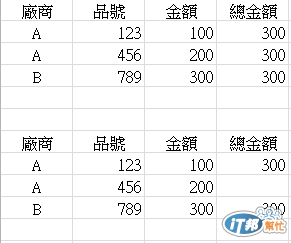#0

## T-SQL 多筆金額統計 顯示方法

``````SELECT 廠商 ,
品號 ,
金額 ,
(SELECT SUM(S.金額)
FROM S.資料表
WHERE S.品號 = 品號
GROUP BY S.廠商) '總金額'
FROM 資料表
``````### 1 個回答

2

try

``````&lt;pre class="c" name="code">
select [廠商],[品號],[金額]
,(case when [群組排序] &lt;> 1 then '' else Convert(varchar,[總金額]) end) as [總金額]
from (
select Row_Number() Over(partition by [廠商] order by [廠商]) as [群組排序]
,*
from ((
select N'A' as [廠商]
, '123' as [品號]
, 100 as [金額]
, 100 as [總金額]
)union(
select N'A' as [廠商]
, '456' as [品號]
, 200 as [金額]
, 300 as [總金額]
)union(
select N'B' as [廠商]
, '789' as [品號]
, 300 as [金額]
, 300 as [總金額]
)) as k
) as k
``````
Albert iT邦高手 1 級 ‧ 2016-02-01 23:45:32 檢舉